Скачать презентацию Quadrature-Mirror Filter Bank In many applications a

b06b5b65d381f4730f171fc720977cfe.ppt

• Количество слайдов: 63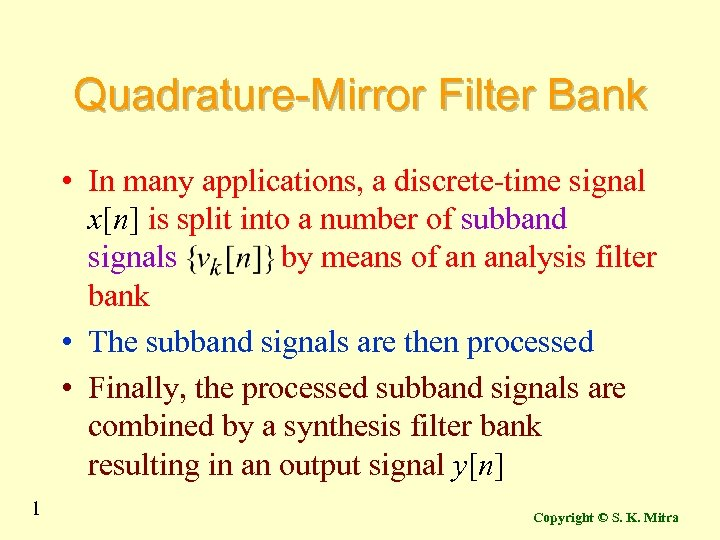Quadrature-Mirror Filter Bank • In many applications, a discrete-time signal x[n] is split into a number of subband signals by means of an analysis filter bank • The subband signals are then processed • Finally, the processed subband signals are combined by a synthesis filter bank resulting in an output signal y[n] 1 Copyright © S. K. Mitra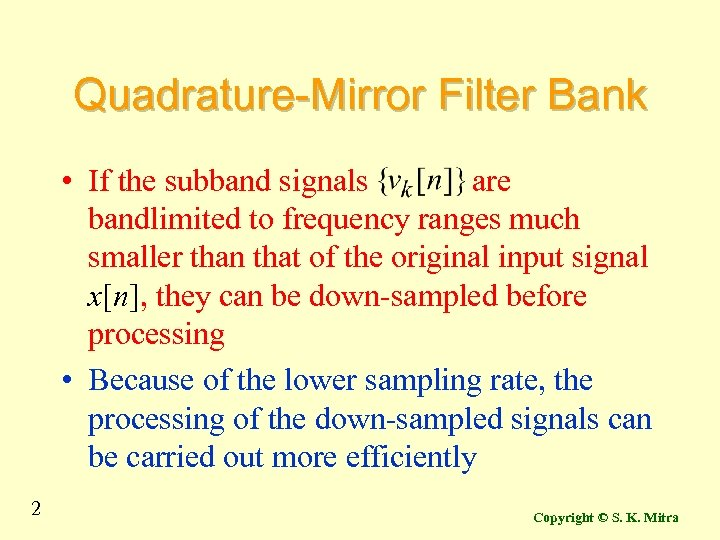Quadrature-Mirror Filter Bank • If the subband signals are bandlimited to frequency ranges much smaller than that of the original input signal x[n], they can be down-sampled before processing • Because of the lower sampling rate, the processing of the down-sampled signals can be carried out more efficiently 2 Copyright © S. K. Mitra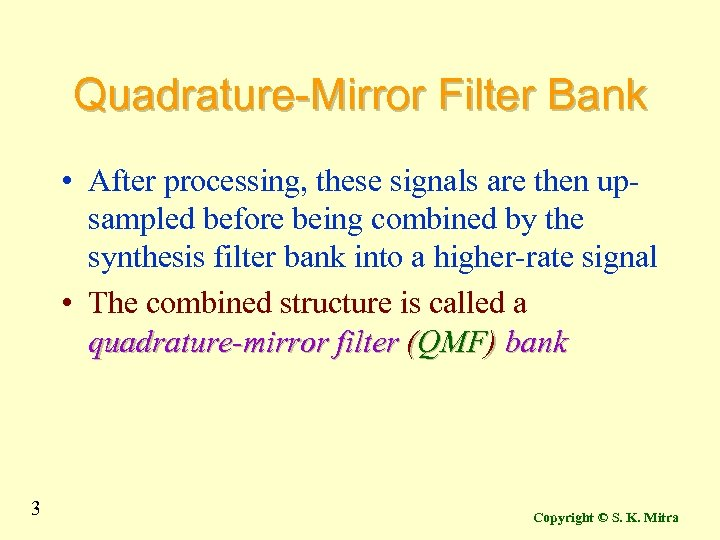Quadrature-Mirror Filter Bank • After processing, these signals are then upsampled before being combined by the synthesis filter bank into a higher-rate signal • The combined structure is called a quadrature-mirror filter (QMF) bank 3 Copyright © S. K. Mitra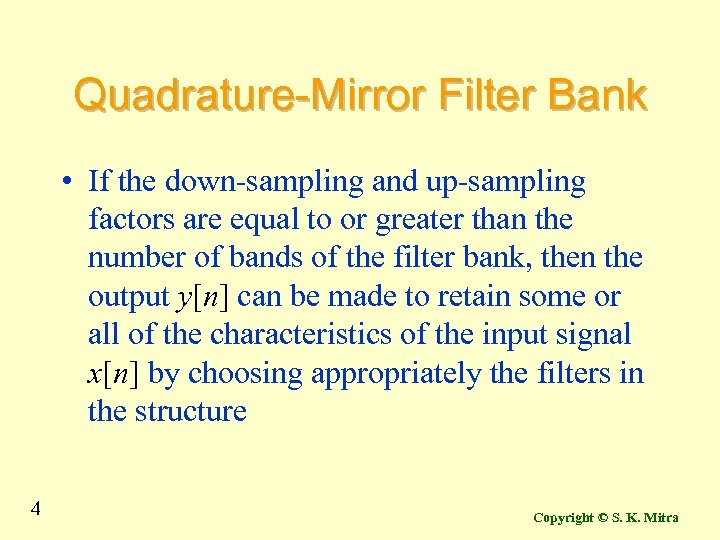Quadrature-Mirror Filter Bank • If the down-sampling and up-sampling factors are equal to or greater than the number of bands of the filter bank, then the output y[n] can be made to retain some or all of the characteristics of the input signal x[n] by choosing appropriately the filters in the structure 4 Copyright © S. K. Mitra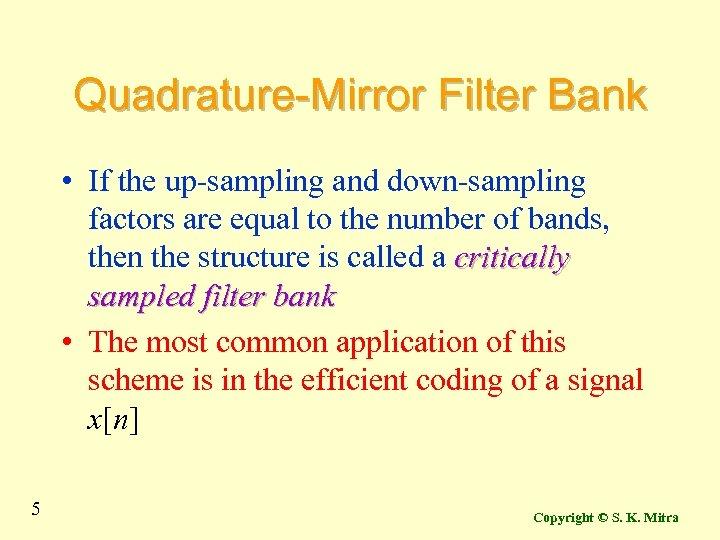Quadrature-Mirror Filter Bank • If the up-sampling and down-sampling factors are equal to the number of bands, then the structure is called a critically sampled filter bank • The most common application of this scheme is in the efficient coding of a signal x[n] 5 Copyright © S. K. Mitra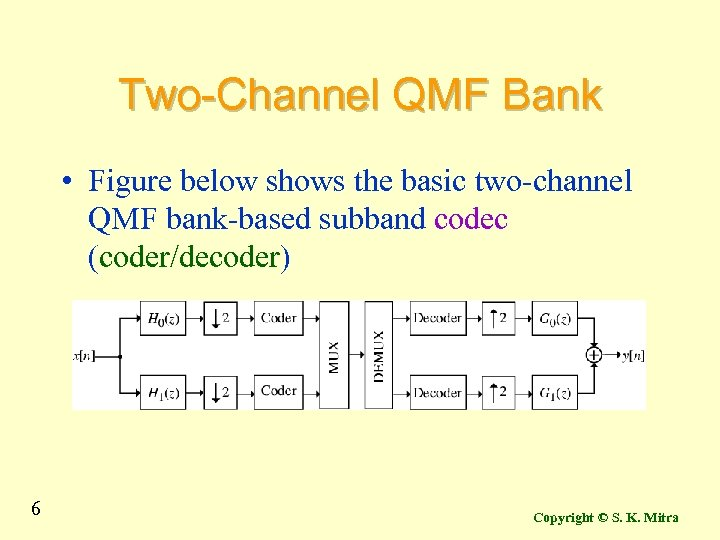Two-Channel QMF Bank • Figure below shows the basic two-channel QMF bank-based subband codec (coder/decoder) 6 Copyright © S. K. Mitra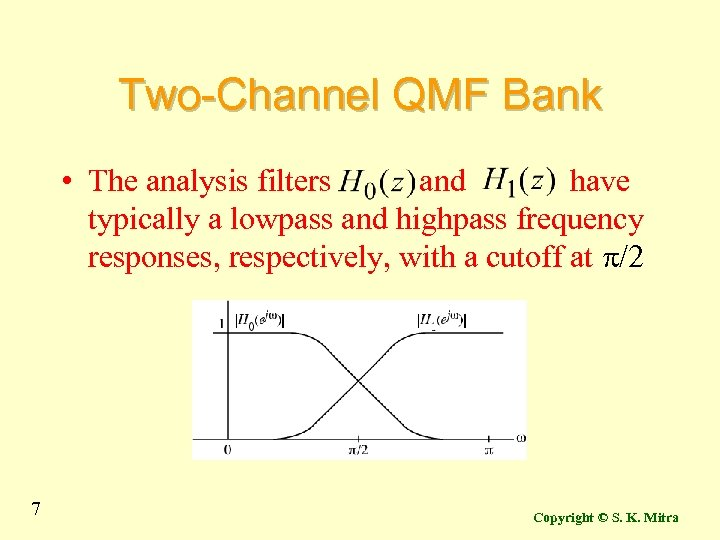Two-Channel QMF Bank • The analysis filters and have typically a lowpass and highpass frequency responses, respectively, with a cutoff at p/2 7 Copyright © S. K. Mitra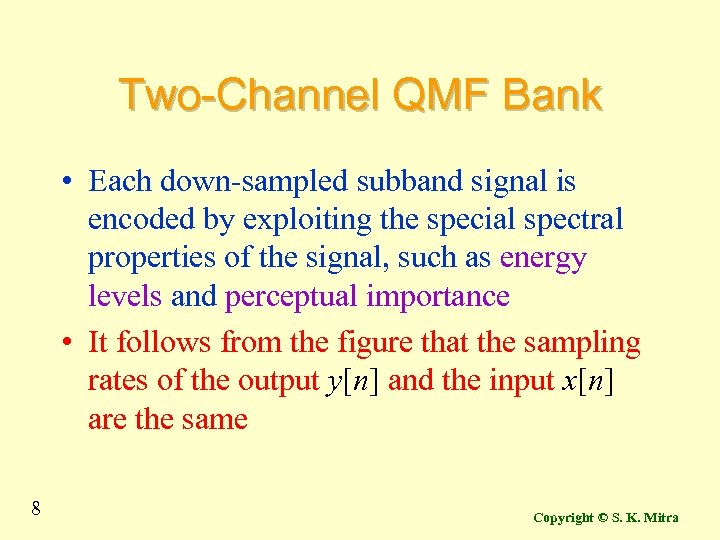Two-Channel QMF Bank • Each down-sampled subband signal is encoded by exploiting the special spectral properties of the signal, such as energy levels and perceptual importance • It follows from the figure that the sampling rates of the output y[n] and the input x[n] are the same 8 Copyright © S. K. Mitra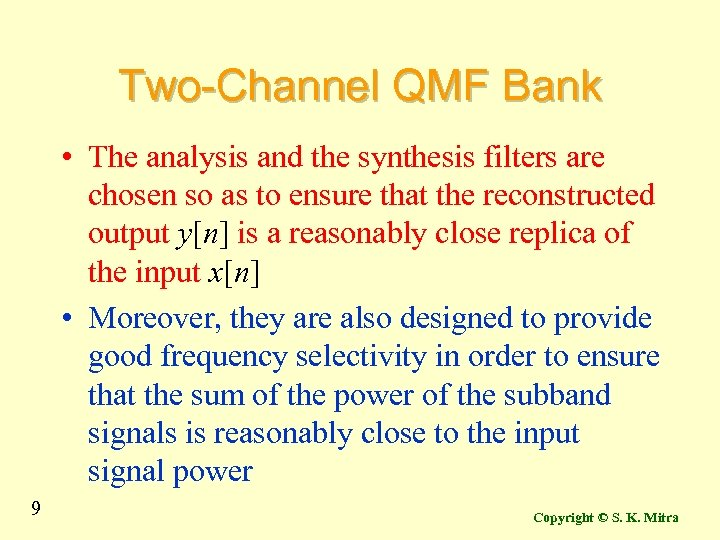Two-Channel QMF Bank • The analysis and the synthesis filters are chosen so as to ensure that the reconstructed output y[n] is a reasonably close replica of the input x[n] • Moreover, they are also designed to provide good frequency selectivity in order to ensure that the sum of the power of the subband signals is reasonably close to the input signal power 9 Copyright © S. K. Mitra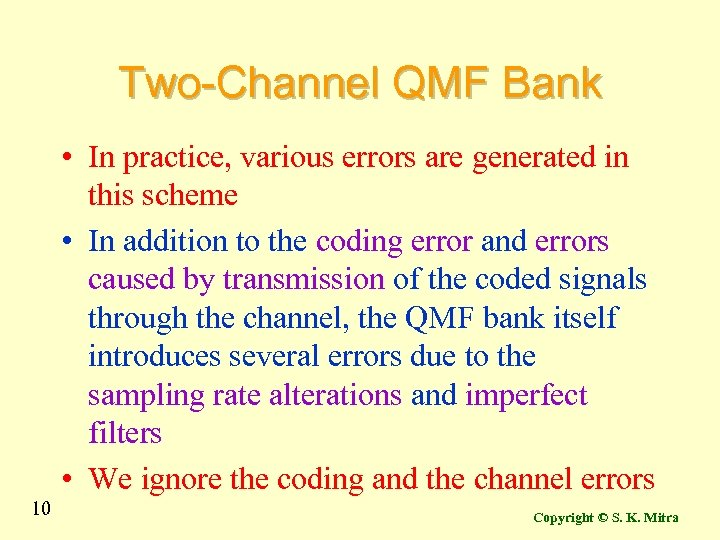Two-Channel QMF Bank • In practice, various errors are generated in this scheme • In addition to the coding error and errors caused by transmission of the coded signals through the channel, the QMF bank itself introduces several errors due to the sampling rate alterations and imperfect filters • We ignore the coding and the channel errors 10 Copyright © S. K. Mitra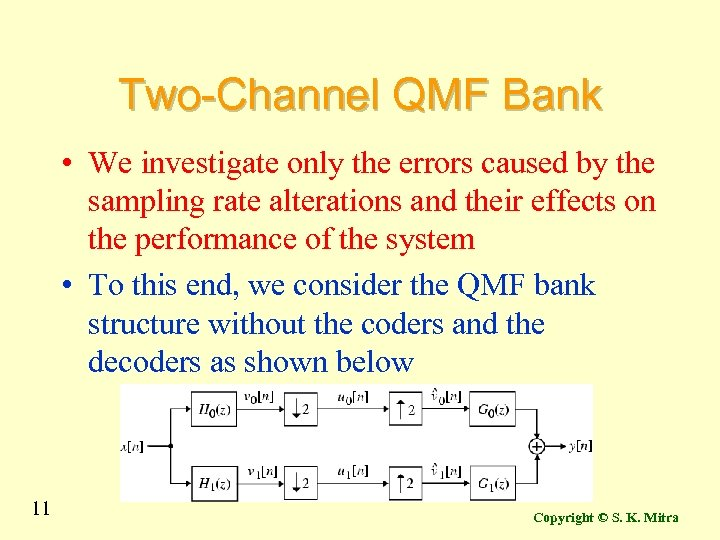Two-Channel QMF Bank • We investigate only the errors caused by the sampling rate alterations and their effects on the performance of the system • To this end, we consider the QMF bank structure without the coders and the decoders as shown below 11 Copyright © S. K. Mitra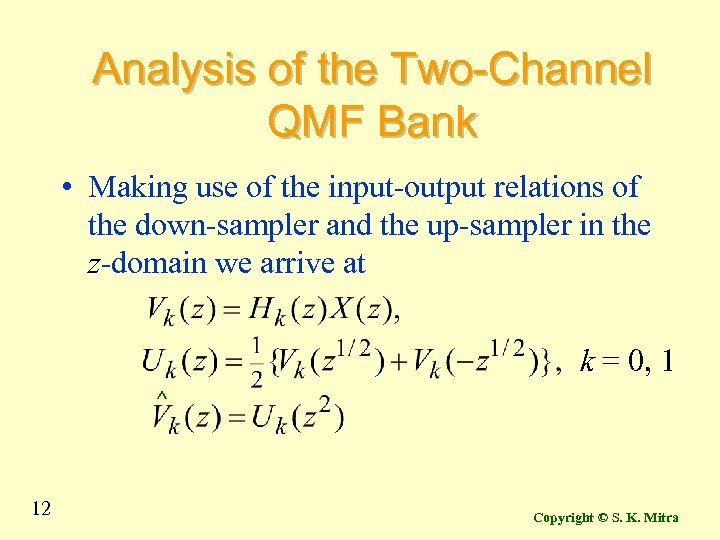Analysis of the Two-Channel QMF Bank • Making use of the input-output relations of the down-sampler and the up-sampler in the z-domain we arrive at k = 0, 1 ^ 12 Copyright © S. K. Mitra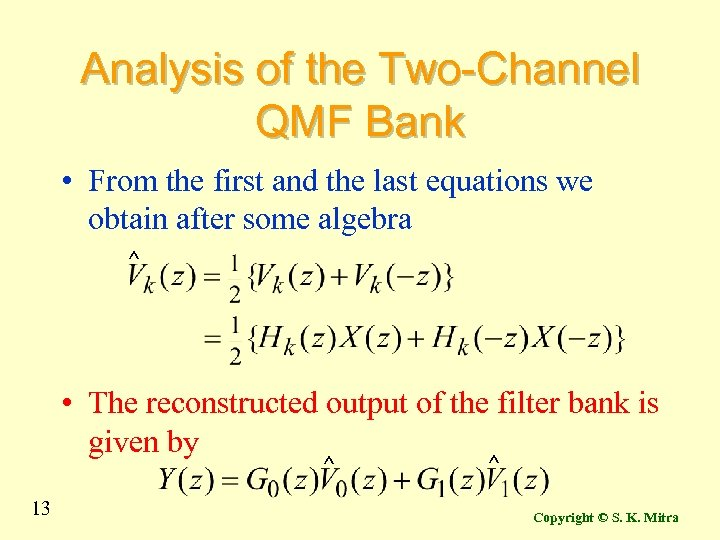Analysis of the Two-Channel QMF Bank • From the first and the last equations we obtain after some algebra ^ • The reconstructed output of the filter bank is given by ^ 13 ^ Copyright © S. K. Mitra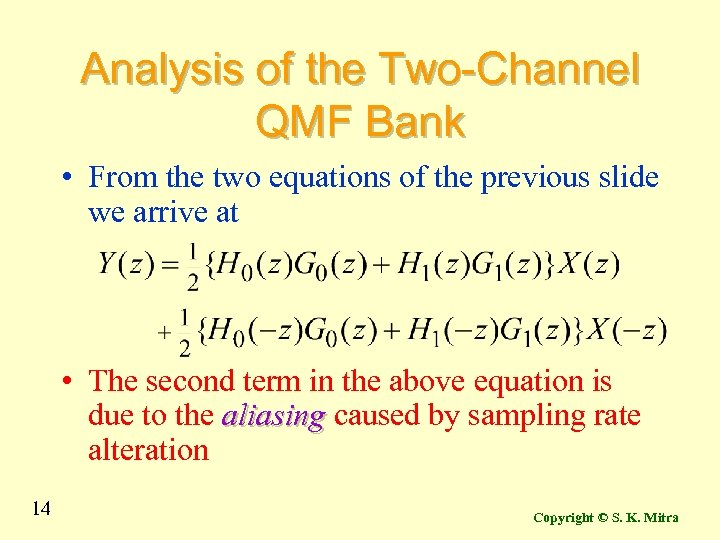Analysis of the Two-Channel QMF Bank • From the two equations of the previous slide we arrive at • The second term in the above equation is due to the aliasing caused by sampling rate alteration 14 Copyright © S. K. Mitra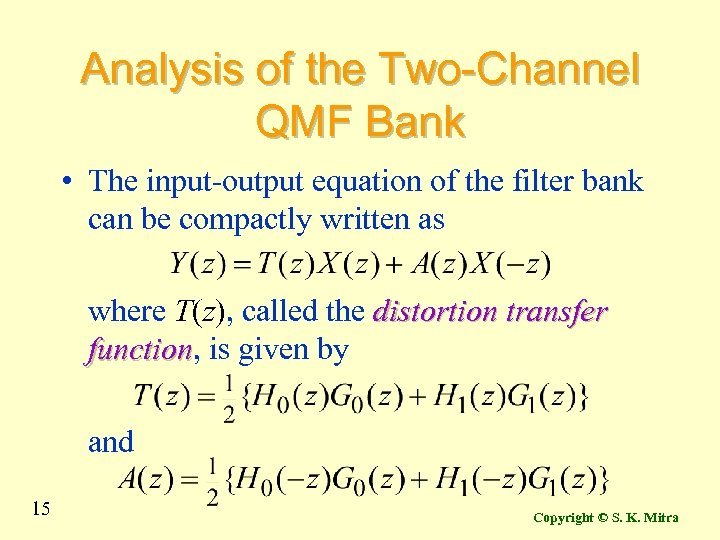Analysis of the Two-Channel QMF Bank • The input-output equation of the filter bank can be compactly written as where T(z), called the distortion transfer function, is given by function and 15 Copyright © S. K. Mitra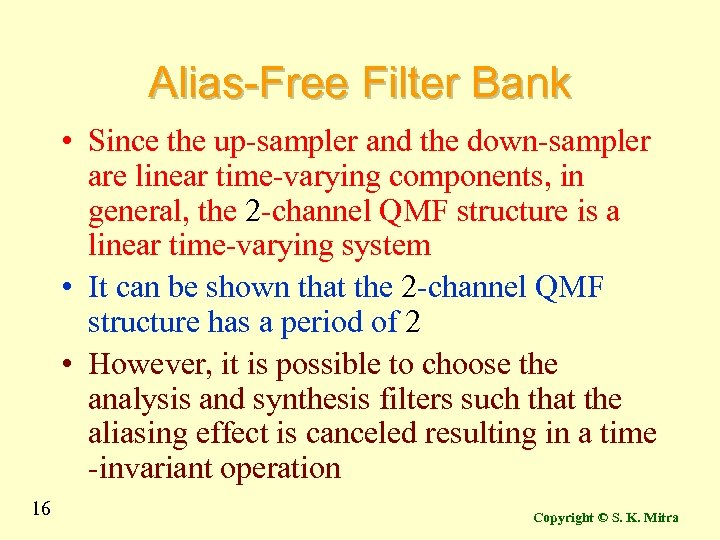Alias-Free Filter Bank • Since the up-sampler and the down-sampler are linear time-varying components, in general, the 2 -channel QMF structure is a linear time-varying system • It can be shown that the 2 -channel QMF structure has a period of 2 • However, it is possible to choose the analysis and synthesis filters such that the aliasing effect is canceled resulting in a time -invariant operation 16 Copyright © S. K. Mitra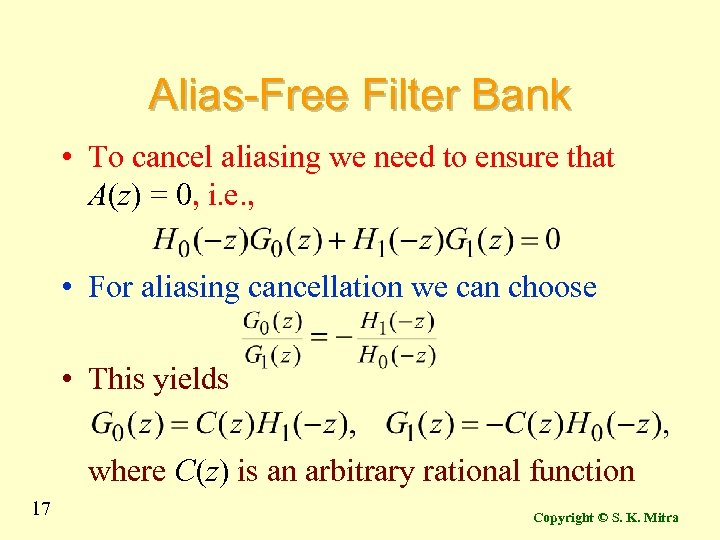Alias-Free Filter Bank • To cancel aliasing we need to ensure that A(z) = 0, i. e. , • For aliasing cancellation we can choose • This yields where C(z) is an arbitrary rational function 17 Copyright © S. K. Mitra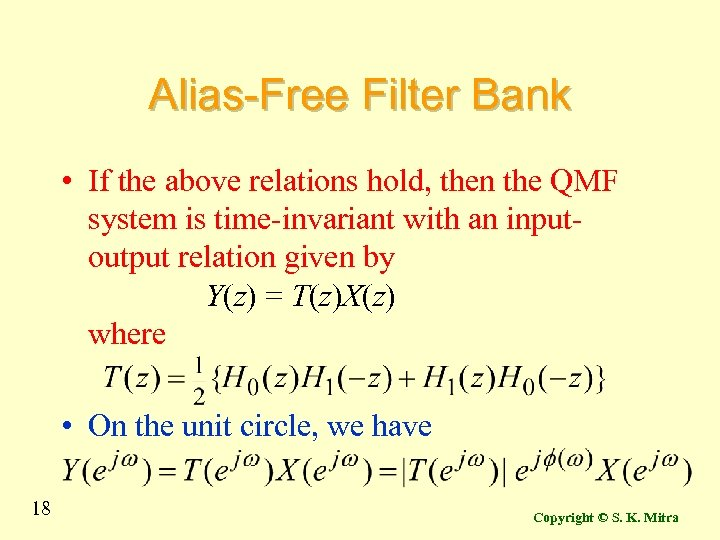Alias-Free Filter Bank • If the above relations hold, then the QMF system is time-invariant with an inputoutput relation given by Y(z) = T(z)X(z) where • On the unit circle, we have 18 Copyright © S. K. Mitra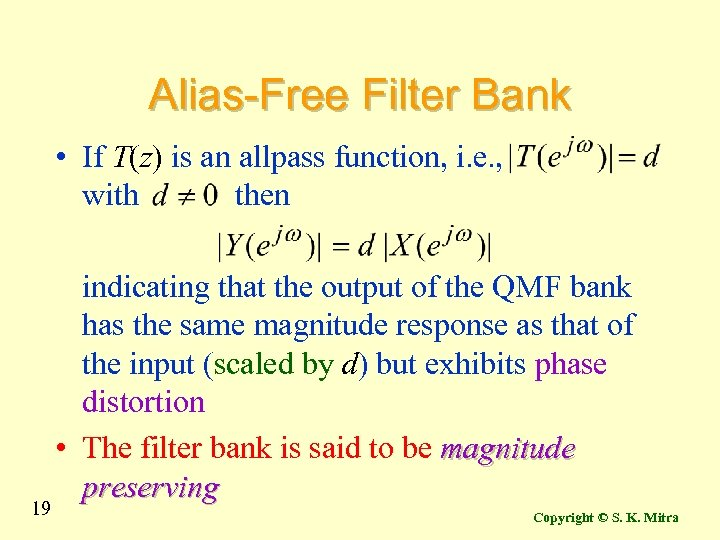Alias-Free Filter Bank • If T(z) is an allpass function, i. e. , with then 19 indicating that the output of the QMF bank has the same magnitude response as that of the input (scaled by d) but exhibits phase distortion • The filter bank is said to be magnitude preserving Copyright © S. K. Mitra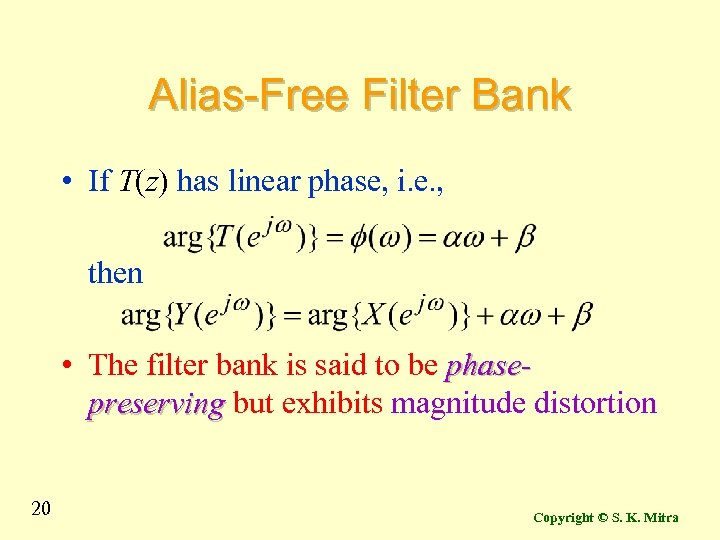Alias-Free Filter Bank • If T(z) has linear phase, i. e. , then • The filter bank is said to be phasepreserving but exhibits magnitude distortion 20 Copyright © S. K. Mitra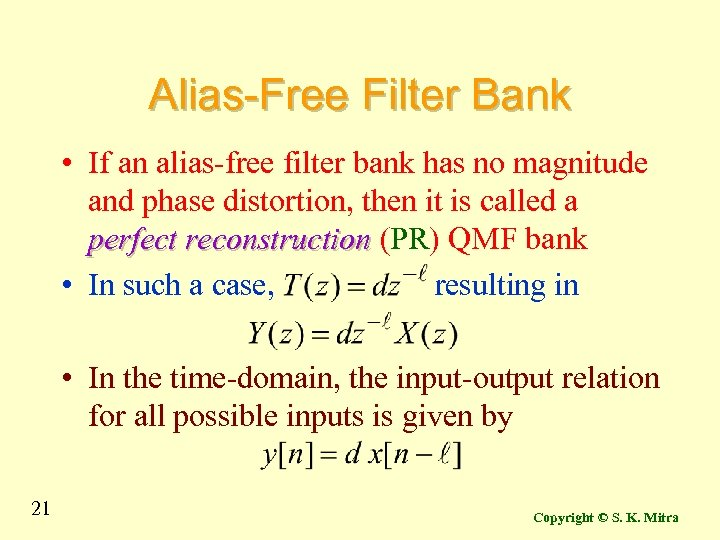Alias-Free Filter Bank • If an alias-free filter bank has no magnitude and phase distortion, then it is called a perfect reconstruction (PR) QMF bank • In such a case, resulting in • In the time-domain, the input-output relation for all possible inputs is given by 21 Copyright © S. K. Mitra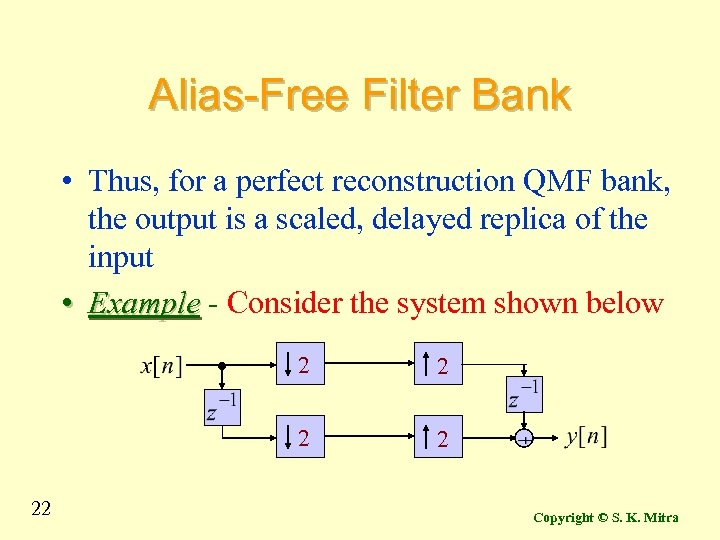Alias-Free Filter Bank • Thus, for a perfect reconstruction QMF bank, the output is a scaled, delayed replica of the input • Example - Consider the system shown below 2 2 2 Copyright © S. K. Mitra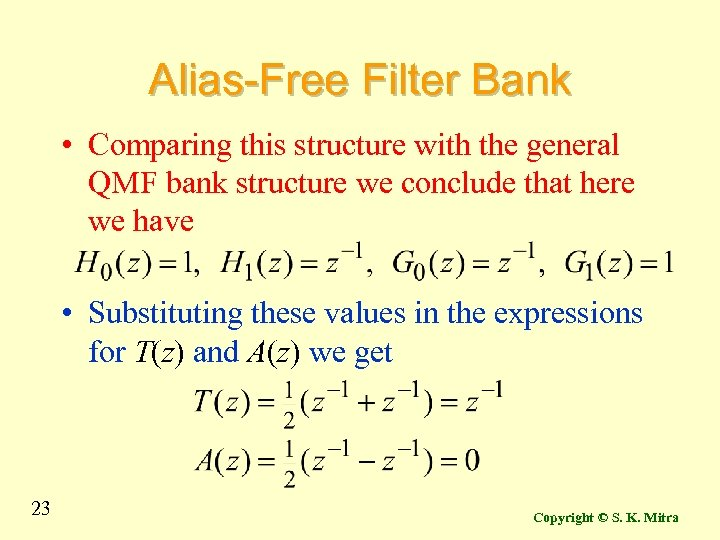Alias-Free Filter Bank • Comparing this structure with the general QMF bank structure we conclude that here we have • Substituting these values in the expressions for T(z) and A(z) we get 23 Copyright © S. K. Mitra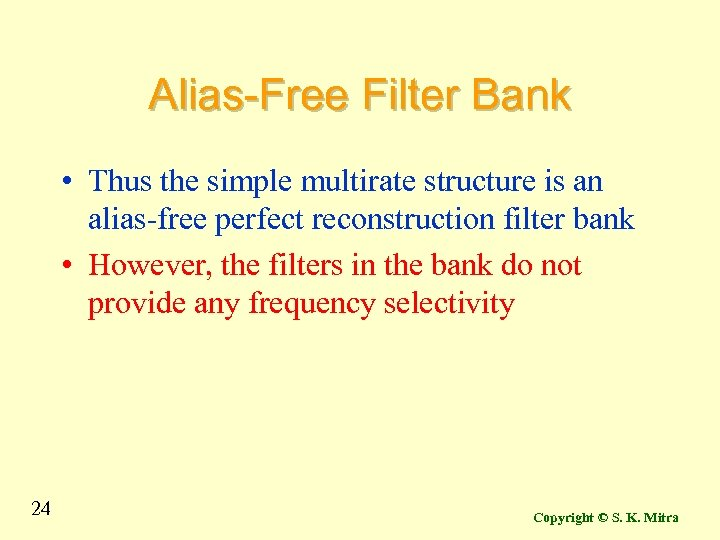Alias-Free Filter Bank • Thus the simple multirate structure is an alias-free perfect reconstruction filter bank • However, the filters in the bank do not provide any frequency selectivity 24 Copyright © S. K. Mitra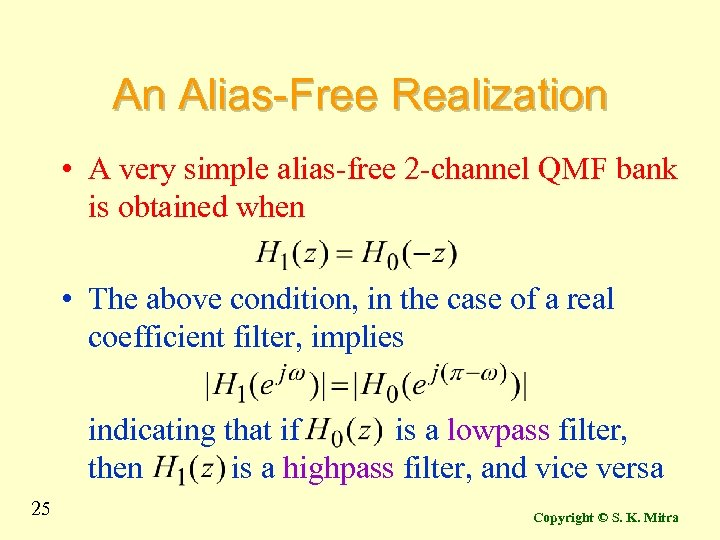An Alias-Free Realization • A very simple alias-free 2 -channel QMF bank is obtained when • The above condition, in the case of a real coefficient filter, implies indicating that if is a lowpass filter, then is a highpass filter, and vice versa 25 Copyright © S. K. Mitra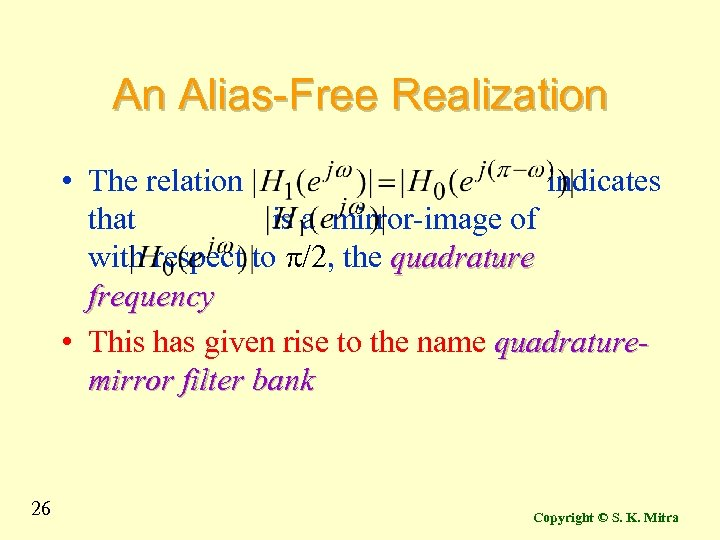An Alias-Free Realization • The relation indicates that is a mirror-image of with respect to p/2, the quadrature frequency • This has given rise to the name quadraturemirror filter bank 26 Copyright © S. K. Mitra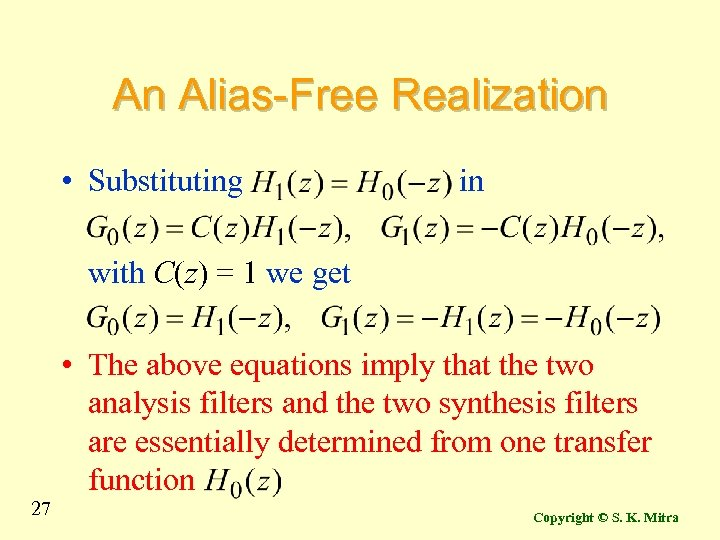An Alias-Free Realization • Substituting in with C(z) = 1 we get • The above equations imply that the two analysis filters and the two synthesis filters are essentially determined from one transfer function 27 Copyright © S. K. Mitra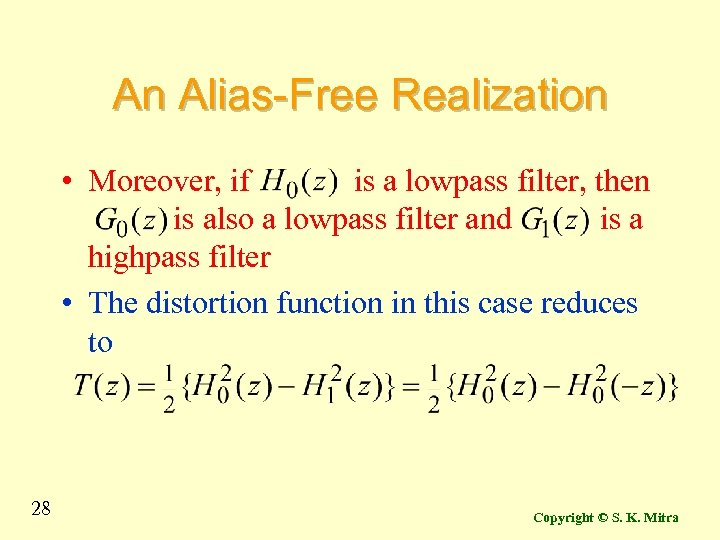An Alias-Free Realization • Moreover, if is a lowpass filter, then is also a lowpass filter and is a highpass filter • The distortion function in this case reduces to 28 Copyright © S. K. Mitra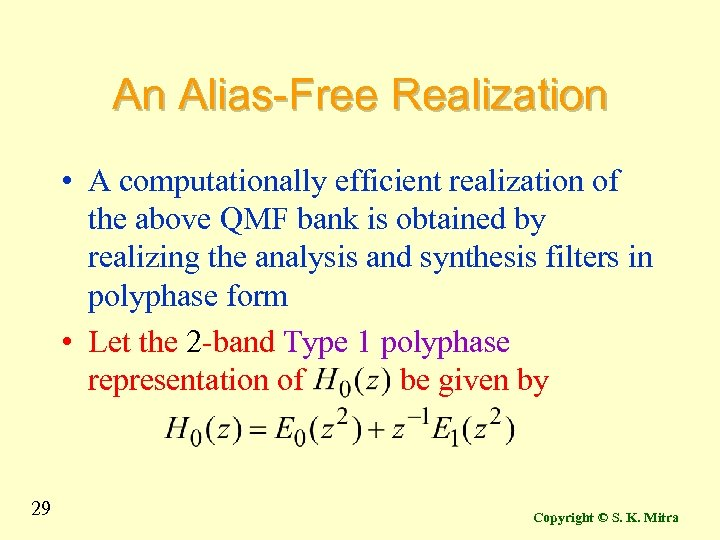An Alias-Free Realization • A computationally efficient realization of the above QMF bank is obtained by realizing the analysis and synthesis filters in polyphase form • Let the 2 -band Type 1 polyphase representation of be given by 29 Copyright © S. K. Mitra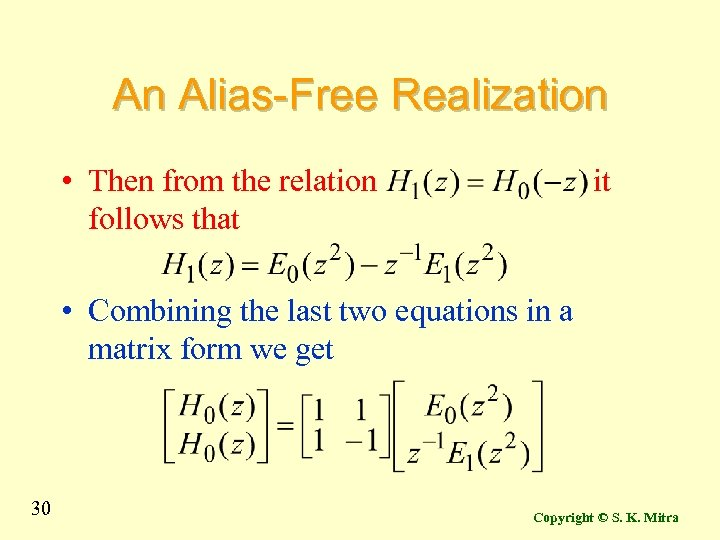An Alias-Free Realization • Then from the relation follows that it • Combining the last two equations in a matrix form we get 30 Copyright © S. K. Mitra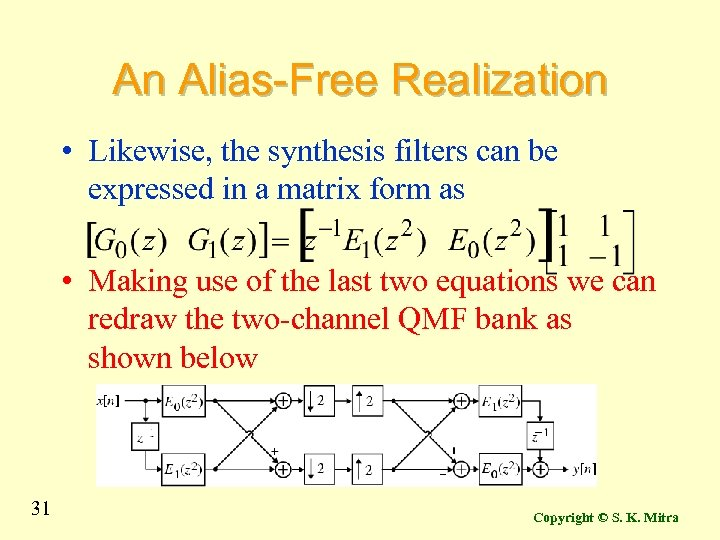An Alias-Free Realization • Likewise, the synthesis filters can be expressed in a matrix form as • Making use of the last two equations we can redraw the two-channel QMF bank as shown below 31 Copyright © S. K. Mitra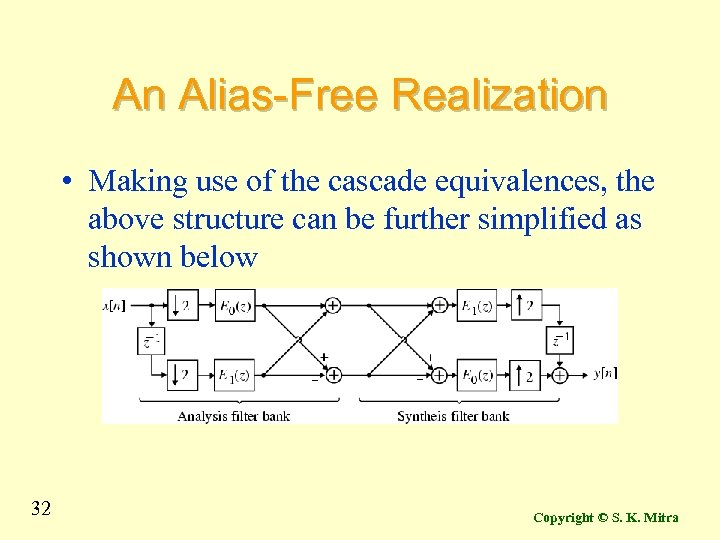An Alias-Free Realization • Making use of the cascade equivalences, the above structure can be further simplified as shown below 32 Copyright © S. K. Mitra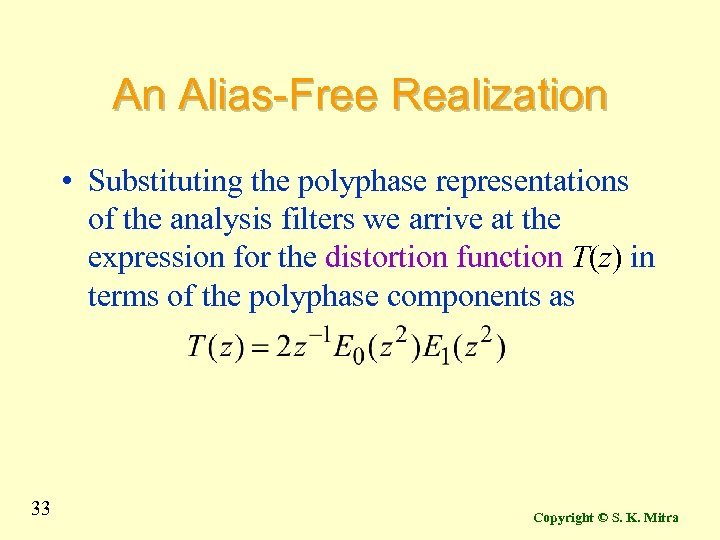An Alias-Free Realization • Substituting the polyphase representations of the analysis filters we arrive at the expression for the distortion function T(z) in terms of the polyphase components as 33 Copyright © S. K. Mitra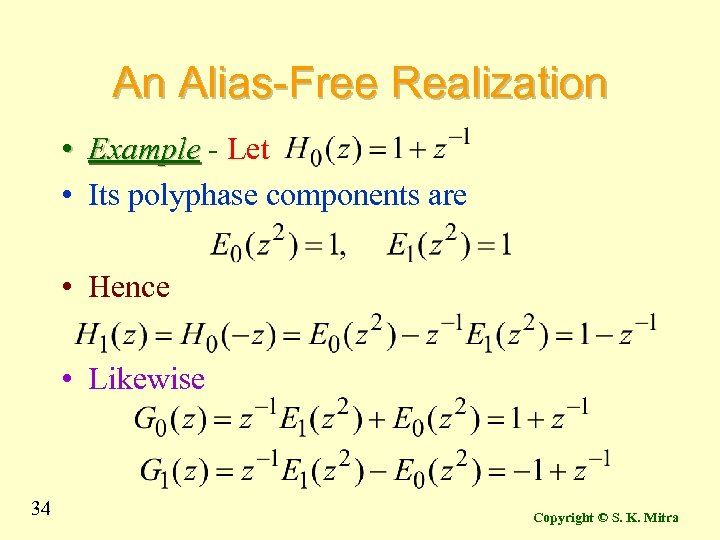An Alias-Free Realization • Example - Let • Its polyphase components are • Hence • Likewise 34 Copyright © S. K. Mitra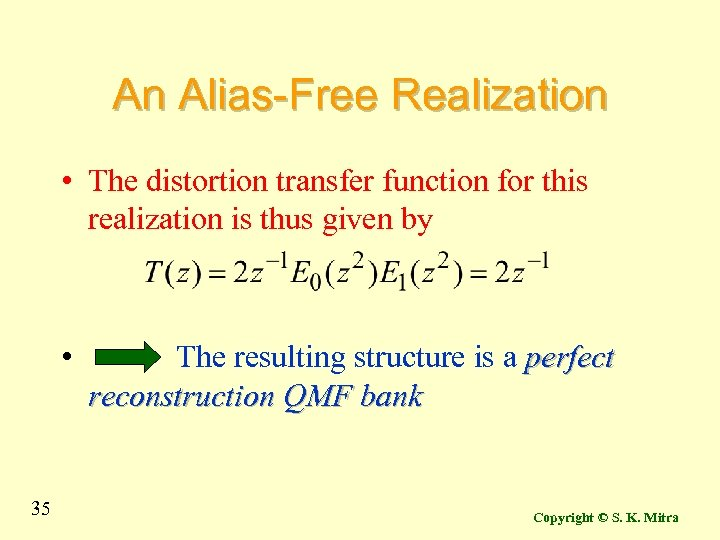An Alias-Free Realization • The distortion transfer function for this realization is thus given by • 35 The resulting structure is a perfect reconstruction QMF bank Copyright © S. K. Mitra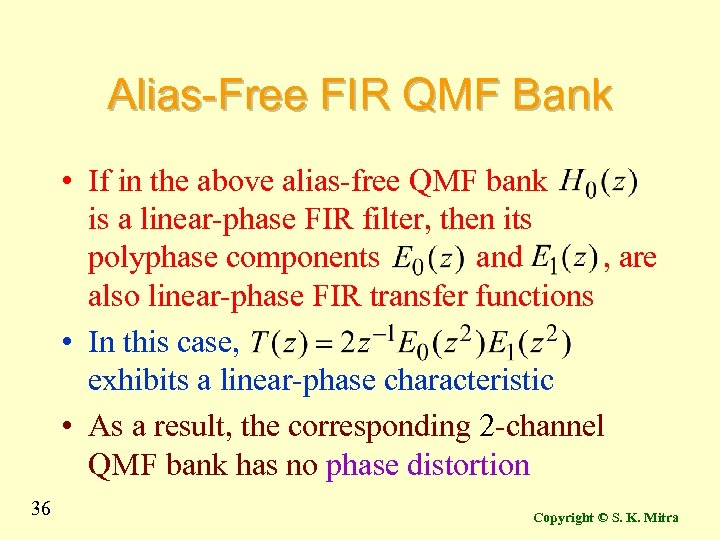Alias-Free FIR QMF Bank • If in the above alias-free QMF bank is a linear-phase FIR filter, then its polyphase components and , are also linear-phase FIR transfer functions • In this case, exhibits a linear-phase characteristic • As a result, the corresponding 2 -channel QMF bank has no phase distortion 36 Copyright © S. K. Mitra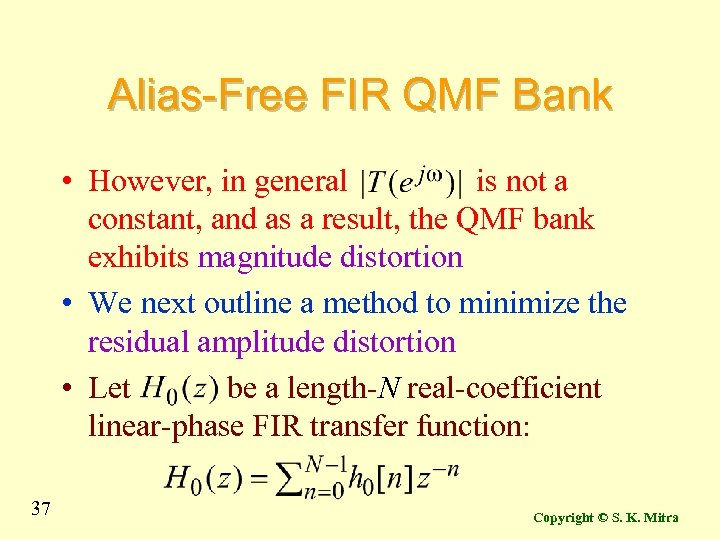Alias-Free FIR QMF Bank • However, in general is not a constant, and as a result, the QMF bank exhibits magnitude distortion • We next outline a method to minimize the residual amplitude distortion • Let be a length-N real-coefficient linear-phase FIR transfer function: 37 Copyright © S. K. Mitra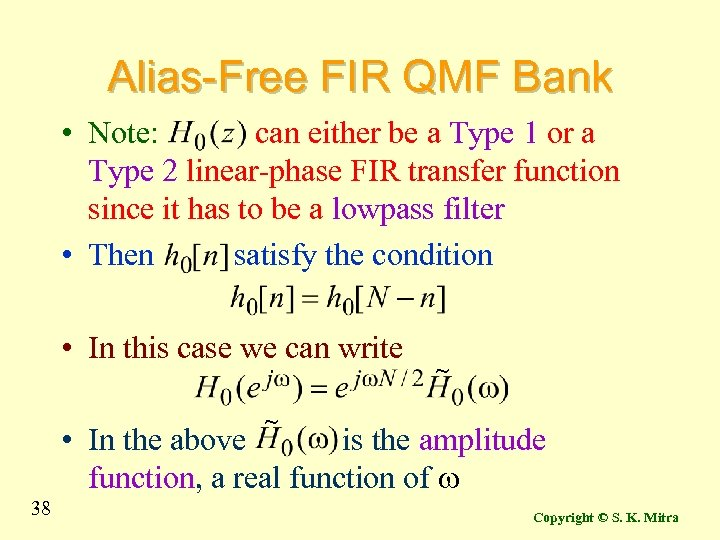Alias-Free FIR QMF Bank • Note: can either be a Type 1 or a Type 2 linear-phase FIR transfer function since it has to be a lowpass filter • Then satisfy the condition • In this case we can write ~ ~ • In the above is the amplitude function, a real function of w 38 Copyright © S. K. Mitra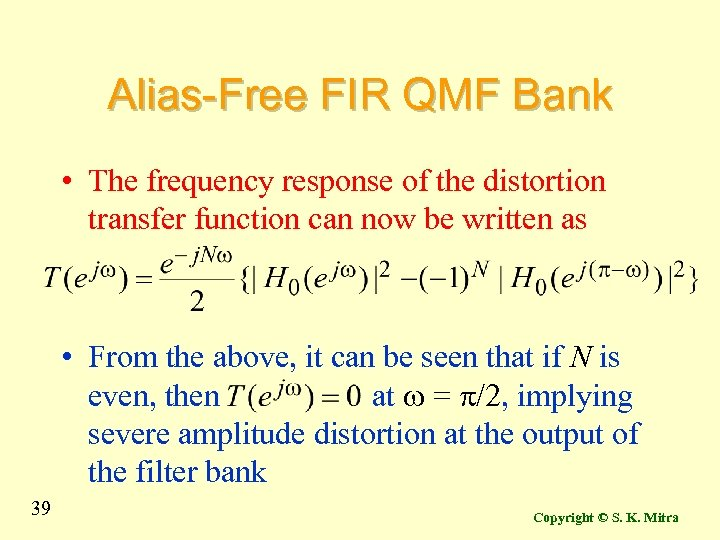Alias-Free FIR QMF Bank • The frequency response of the distortion transfer function can now be written as • From the above, it can be seen that if N is even, then at w = p/2, implying severe amplitude distortion at the output of the filter bank 39 Copyright © S. K. Mitra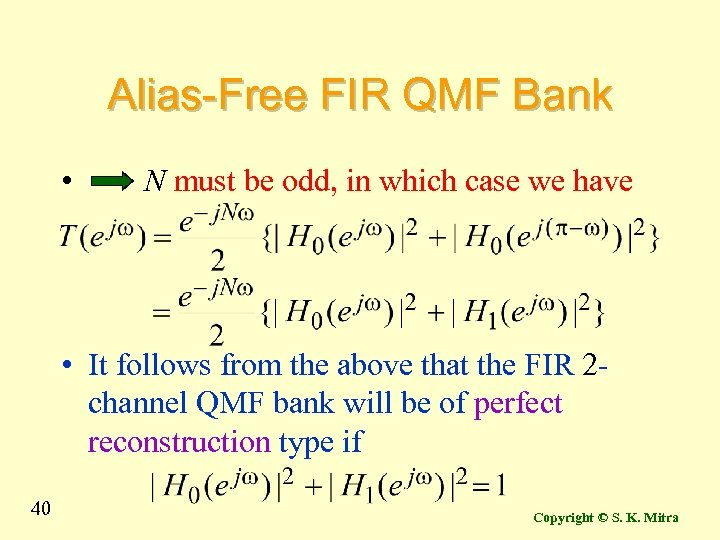Alias-Free FIR QMF Bank • N must be odd, in which case we have • It follows from the above that the FIR 2 channel QMF bank will be of perfect reconstruction type if 40 Copyright © S. K. Mitra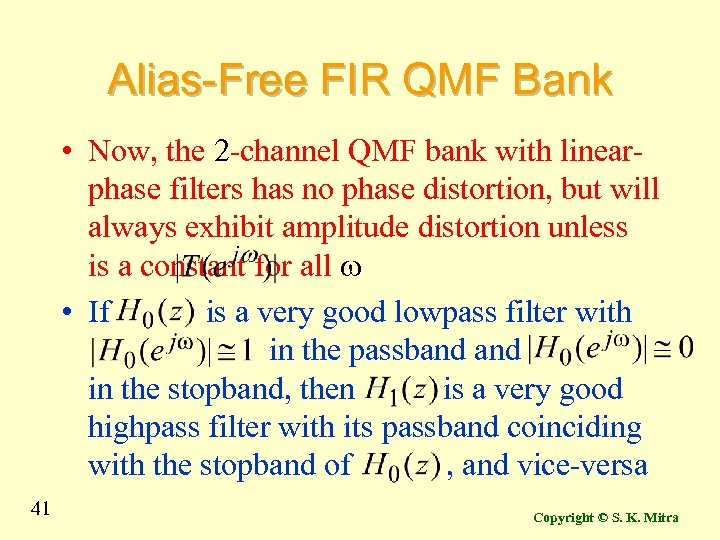Alias-Free FIR QMF Bank • Now, the 2 -channel QMF bank with linearphase filters has no phase distortion, but will always exhibit amplitude distortion unless is a constant for all w • If is a very good lowpass filter with in the passband in the stopband, then is a very good highpass filter with its passband coinciding with the stopband of , and vice-versa 41 Copyright © S. K. Mitra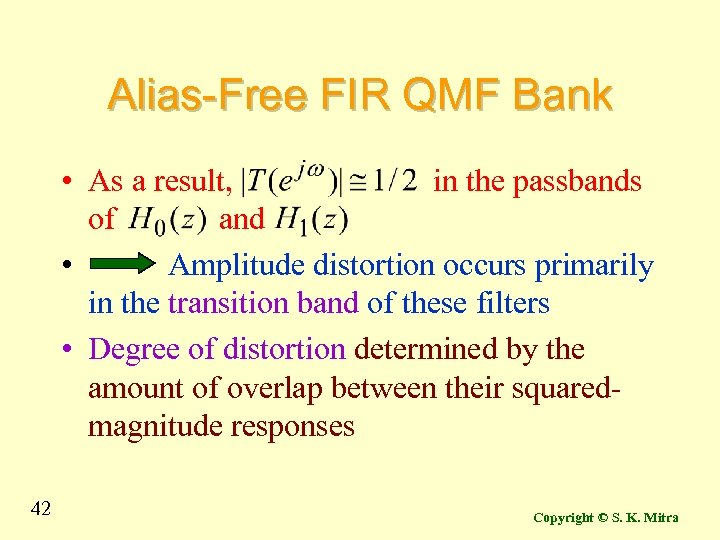Alias-Free FIR QMF Bank • As a result, in the passbands of and • Amplitude distortion occurs primarily in the transition band of these filters • Degree of distortion determined by the amount of overlap between their squaredmagnitude responses 42 Copyright © S. K. Mitra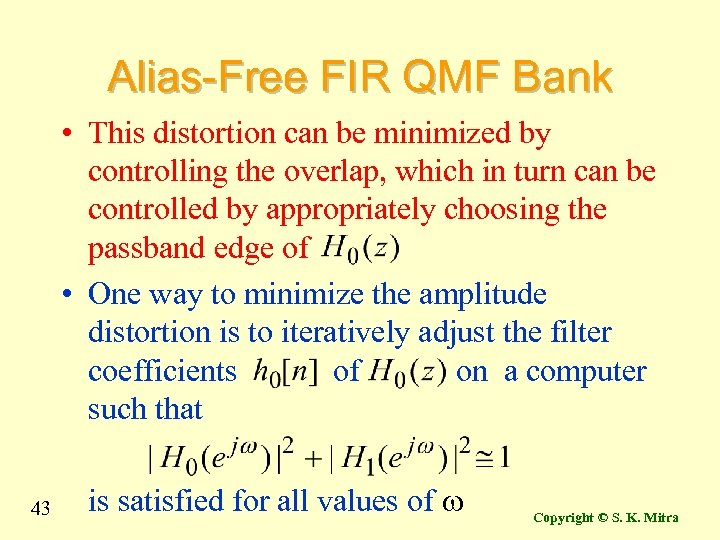Alias-Free FIR QMF Bank • This distortion can be minimized by controlling the overlap, which in turn can be controlled by appropriately choosing the passband edge of • One way to minimize the amplitude distortion is to iteratively adjust the filter coefficients of on a computer such that 43 is satisfied for all values of w Copyright © S. K. Mitra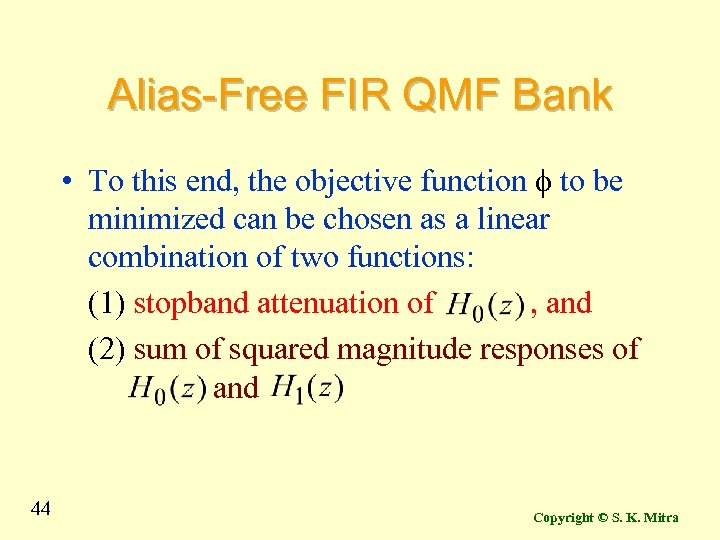Alias-Free FIR QMF Bank • To this end, the objective function f to be minimized can be chosen as a linear combination of two functions: (1) stopband attenuation of , and (2) sum of squared magnitude responses of and 44 Copyright © S. K. Mitra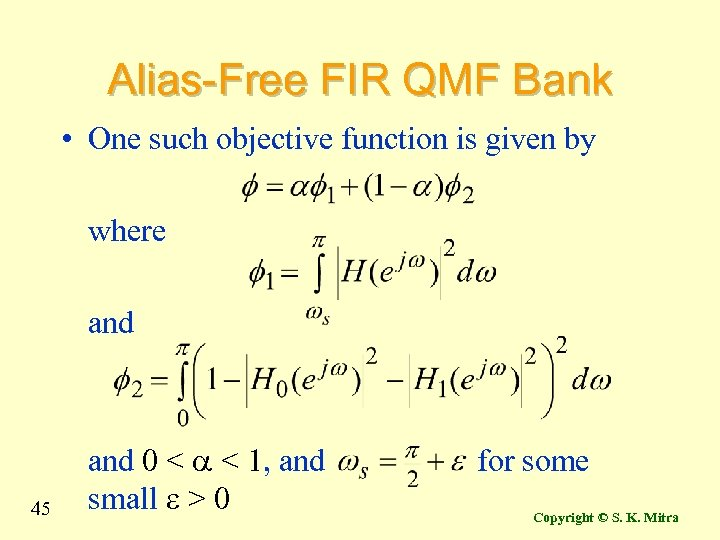Alias-Free FIR QMF Bank • One such objective function is given by where and 45 and 0 < a < 1, and small e > 0 for some Copyright © S. K. Mitra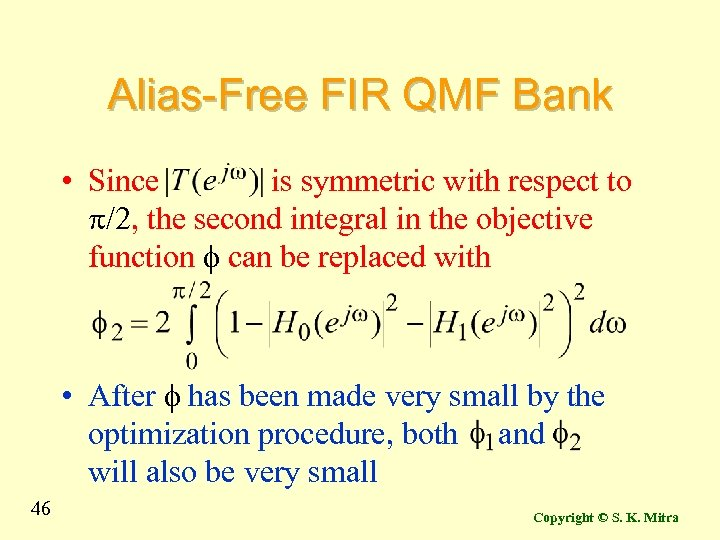Alias-Free FIR QMF Bank • Since is symmetric with respect to p/2, the second integral in the objective function f can be replaced with • After f has been made very small by the optimization procedure, both and will also be very small 46 Copyright © S. K. Mitra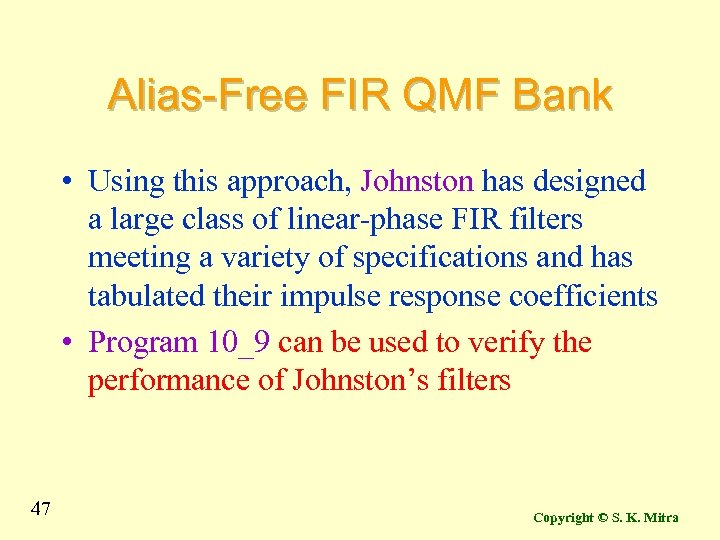Alias-Free FIR QMF Bank • Using this approach, Johnston has designed a large class of linear-phase FIR filters meeting a variety of specifications and has tabulated their impulse response coefficients • Program 10_9 can be used to verify the performance of Johnston’s filters 47 Copyright © S. K. Mitra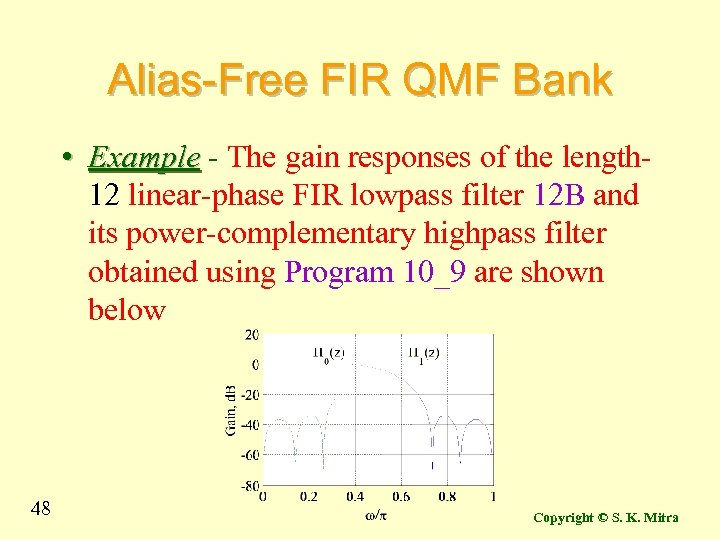Alias-Free FIR QMF Bank • Example - The gain responses of the length 12 linear-phase FIR lowpass filter 12 B and its power-complementary highpass filter obtained using Program 10_9 are shown below 48 Copyright © S. K. Mitra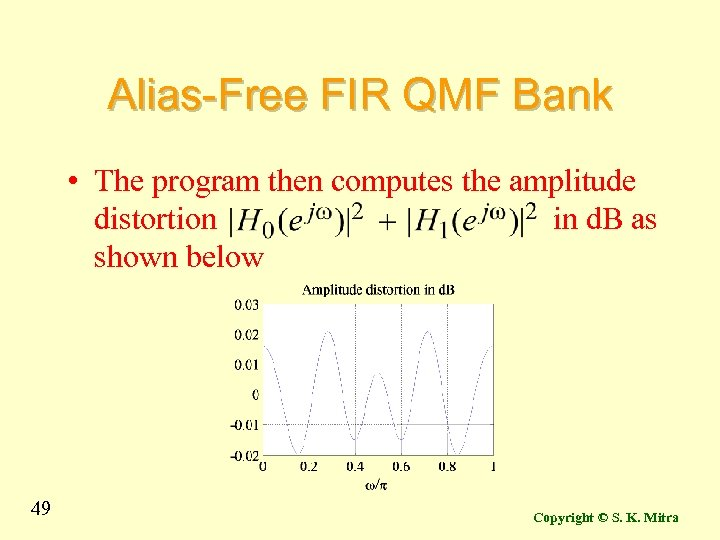Alias-Free FIR QMF Bank • The program then computes the amplitude distortion in d. B as shown below 49 Copyright © S. K. Mitra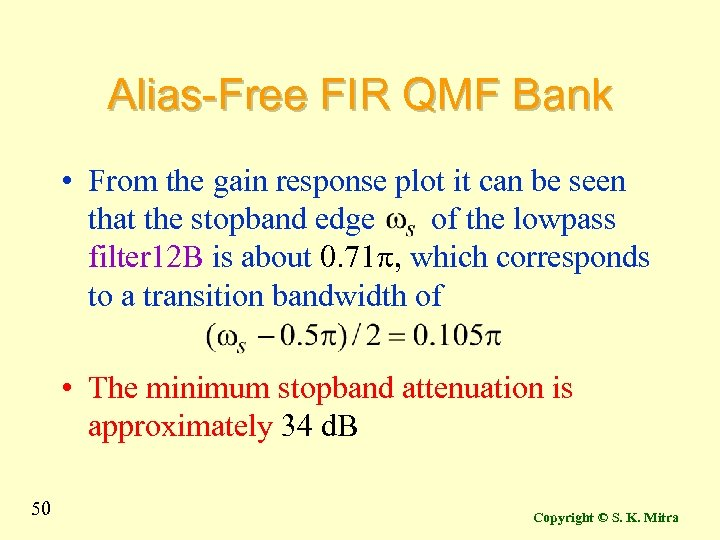Alias-Free FIR QMF Bank • From the gain response plot it can be seen that the stopband edge of the lowpass filter 12 B is about 0. 71 p, which corresponds to a transition bandwidth of • The minimum stopband attenuation is approximately 34 d. B 50 Copyright © S. K. Mitra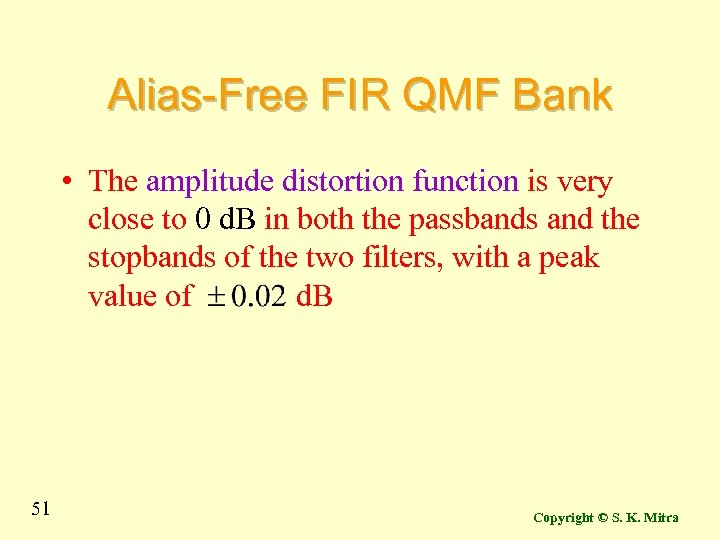Alias-Free FIR QMF Bank • The amplitude distortion function is very close to 0 d. B in both the passbands and the stopbands of the two filters, with a peak value of d. B 51 Copyright © S. K. Mitra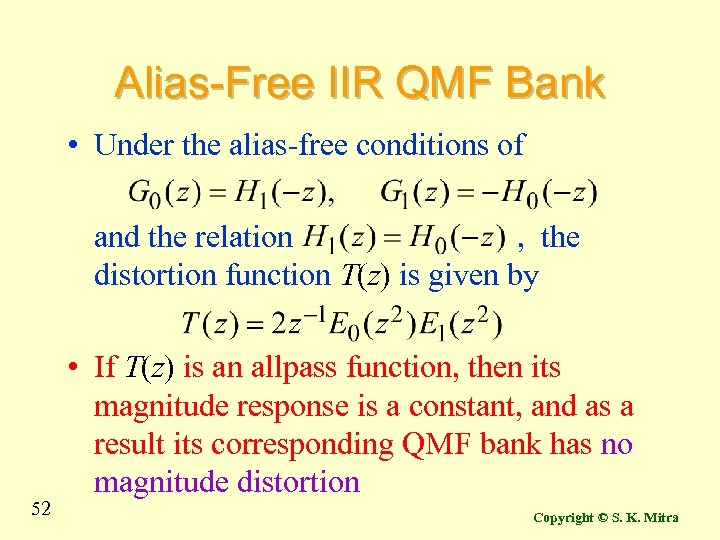Alias-Free IIR QMF Bank • Under the alias-free conditions of and the relation , the distortion function T(z) is given by 52 • If T(z) is an allpass function, then its magnitude response is a constant, and as a result its corresponding QMF bank has no magnitude distortion Copyright © S. K. Mitra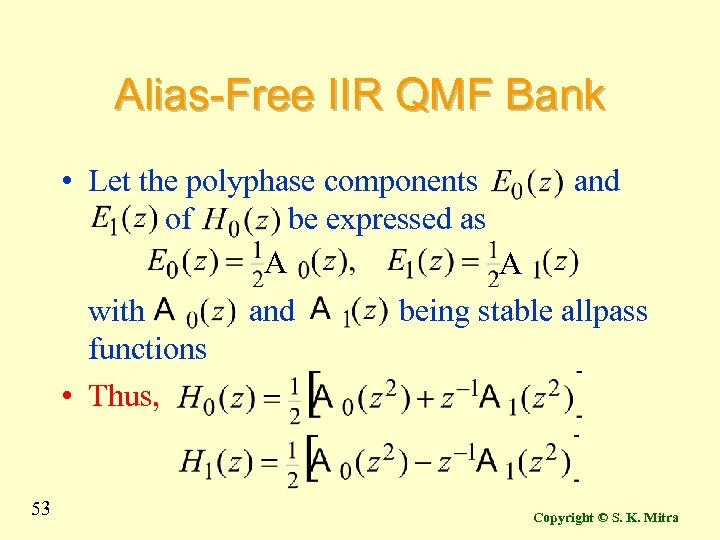Alias-Free IIR QMF Bank • Let the polyphase components and of be expressed as A A with and being stable allpass functions • Thus, 53 Copyright © S. K. Mitra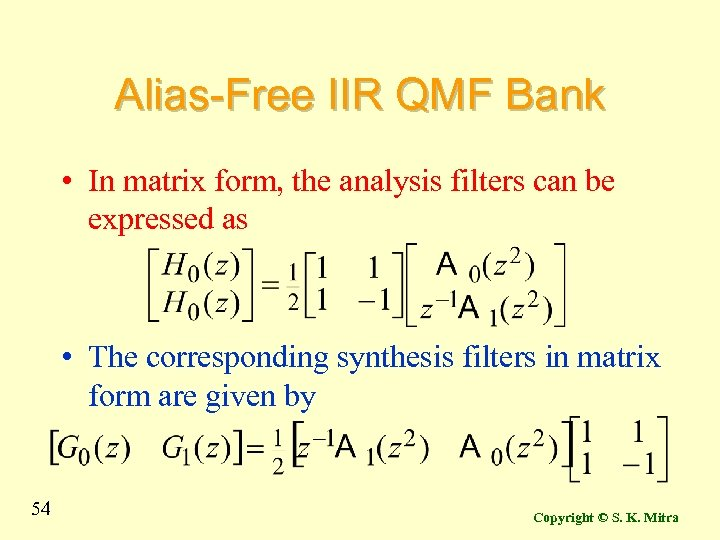Alias-Free IIR QMF Bank • In matrix form, the analysis filters can be expressed as • The corresponding synthesis filters in matrix form are given by 54 Copyright © S. K. Mitra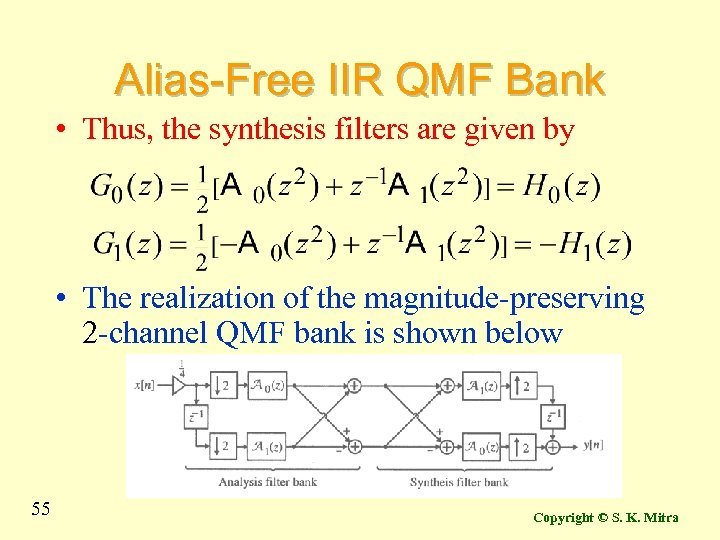Alias-Free IIR QMF Bank • Thus, the synthesis filters are given by • The realization of the magnitude-preserving 2 -channel QMF bank is shown below 55 Copyright © S. K. Mitra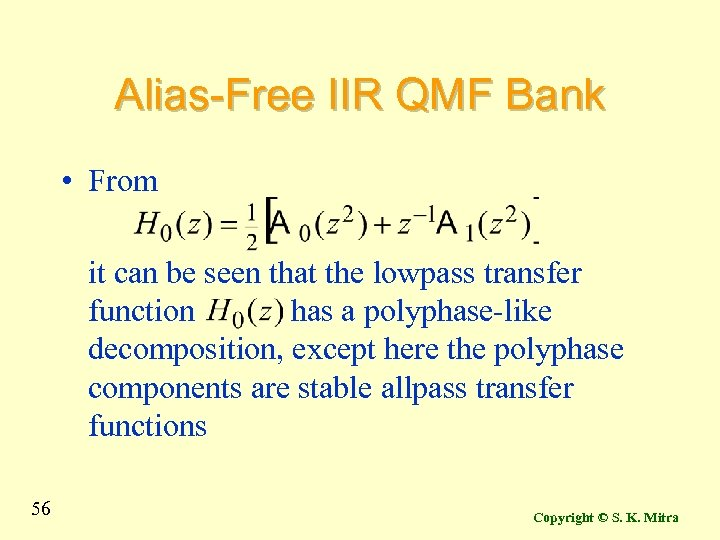Alias-Free IIR QMF Bank • From it can be seen that the lowpass transfer function has a polyphase-like decomposition, except here the polyphase components are stable allpass transfer functions 56 Copyright © S. K. Mitra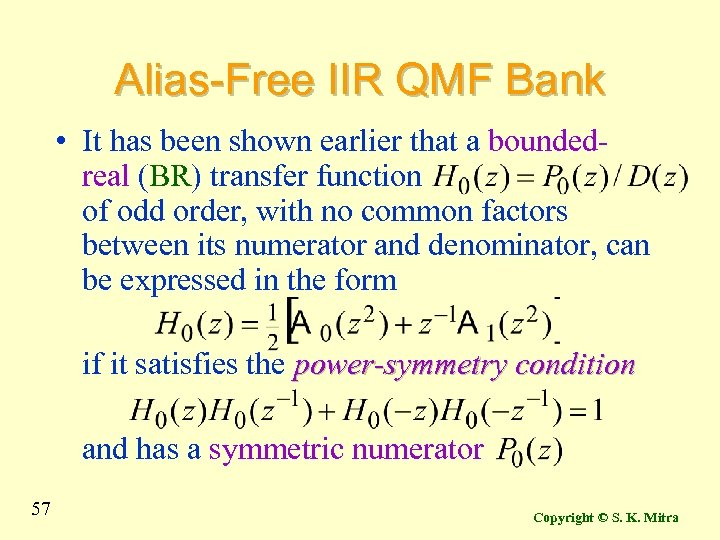Alias-Free IIR QMF Bank • It has been shown earlier that a boundedreal (BR) transfer function of odd order, with no common factors between its numerator and denominator, can be expressed in the form if it satisfies the power-symmetry condition and has a symmetric numerator 57 Copyright © S. K. Mitra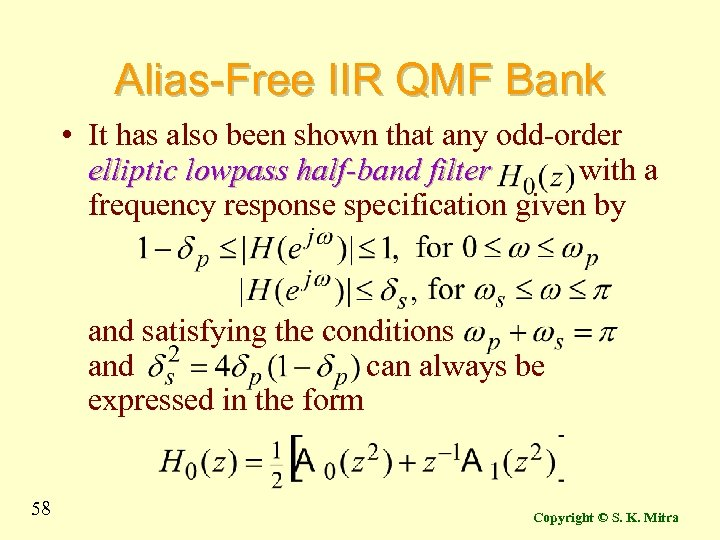Alias-Free IIR QMF Bank • It has also been shown that any odd-order elliptic lowpass half-band filter with a frequency response specification given by and satisfying the conditions and can always be expressed in the form 58 Copyright © S. K. Mitra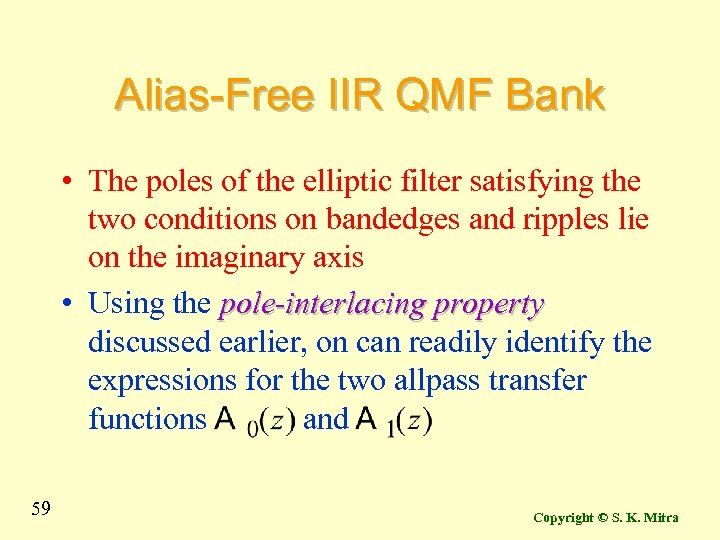Alias-Free IIR QMF Bank • The poles of the elliptic filter satisfying the two conditions on bandedges and ripples lie on the imaginary axis • Using the pole-interlacing property discussed earlier, on can readily identify the expressions for the two allpass transfer functions and 59 Copyright © S. K. Mitra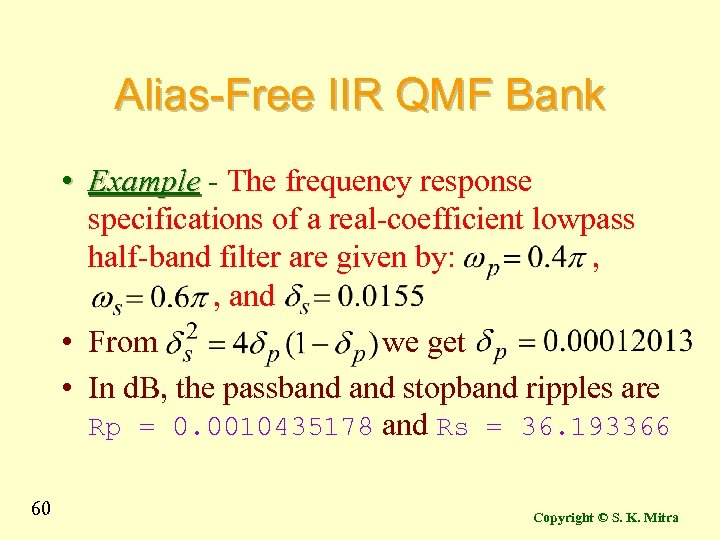Alias-Free IIR QMF Bank • Example - The frequency response specifications of a real-coefficient lowpass half-band filter are given by: , , and • From we get • In d. B, the passband stopband ripples are Rp = 0. 0010435178 and Rs = 36. 193366 60 Copyright © S. K. Mitra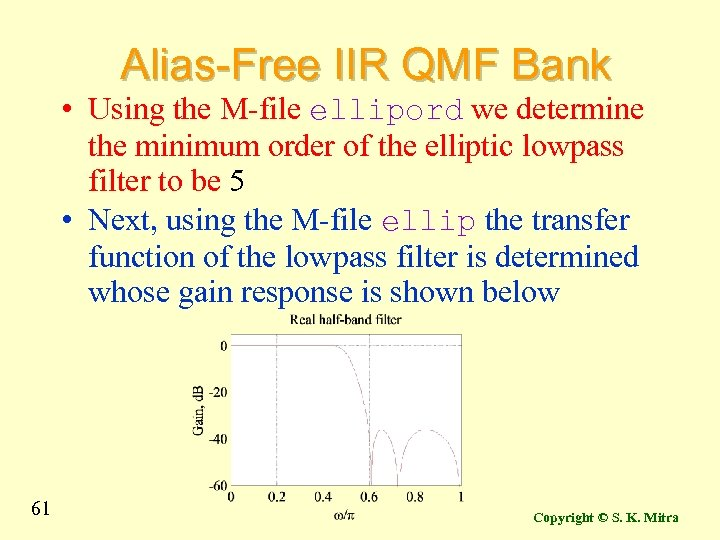Alias-Free IIR QMF Bank • Using the M-file ellipord we determine the minimum order of the elliptic lowpass filter to be 5 • Next, using the M-file ellip the transfer function of the lowpass filter is determined whose gain response is shown below 61 Copyright © S. K. Mitra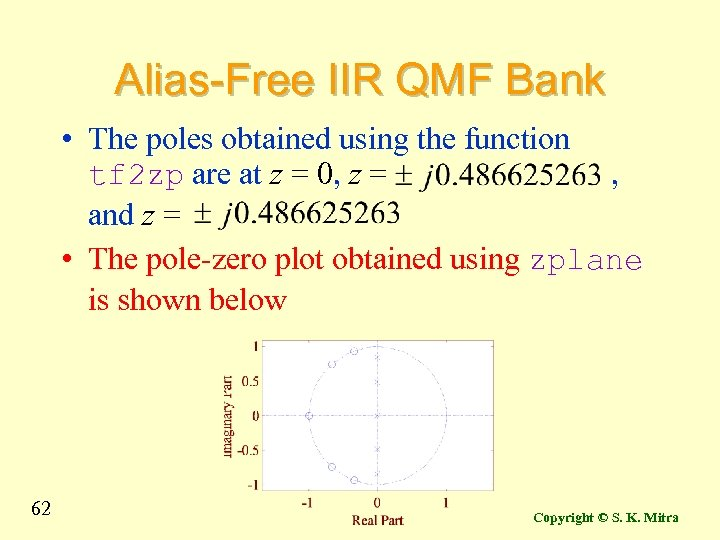Alias-Free IIR QMF Bank • The poles obtained using the function tf 2 zp are at z = 0, z = , and z = • The pole-zero plot obtained using zplane is shown below 62 Copyright © S. K. Mitra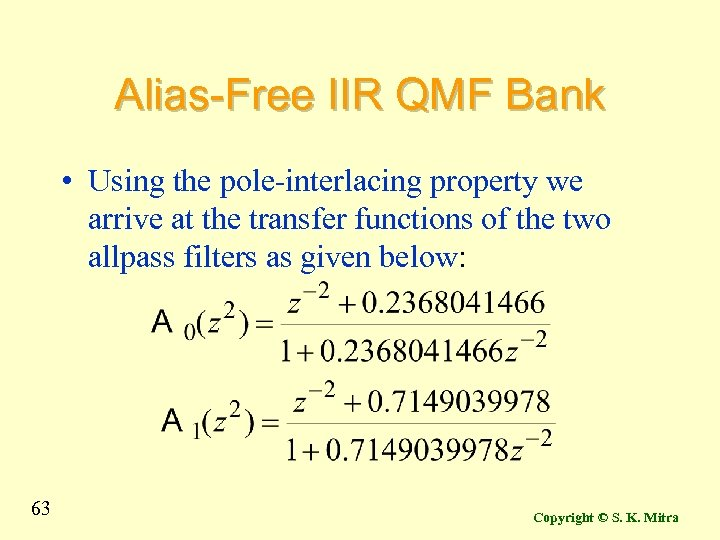Alias-Free IIR QMF Bank • Using the pole-interlacing property we arrive at the transfer functions of the two allpass filters as given below: 63 Copyright © S. K. Mitra